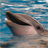# Create a Model

3369
3
03-18-2015 09:15 PMNew Contributor

Hi All,

I need some help to make a model, which will become a geoprocess in Server.

Basically I need to calculate population stats from a point coverage, where each point has the number of people as a value in the 'population' field.

1. I need to draw a polygon on the map which selects all points 'Within' - I have done this

2. Once the selection is made I need to calculate the sum of the values and return that value as the total population in the Area drawn. - This is what I am struggling with

A pretty simple model I am sure, but I have not used Model Maker for a long time as I use FME, and trying to find the tools if problematic, I hope someone out there can assist.

Regards

Tags (2)
1 Solution

Accepted SolutionsOccasional Contributor III

After selection use summary statistics tool which will calculate sum and return the sum in a table.

Further you can use Get Value tool (model only tool) to use this value in next analysis.

Kishor

3 RepliesOccasional Contributor III

After selection use summary statistics tool which will calculate sum and return the sum in a table.

Further you can use Get Value tool (model only tool) to use this value in next analysis.

KishorNew Contributor

Hi Kishore, Thanks very much for this, I just needed pointing in the right direction, all fully operational now, many thanksFrequent Contributor II

Dilip,

Doesn't the Chart Widget have this functionality straight out of the box?

Regards

Gigzy